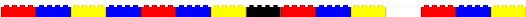# Random Hall Lego Robotics Seminar## Common IC Commands

#### INPUT / OUTPUT:

```   motor(m, p); -   Turn on motor #m at power level p.
p can range from -100 (full reverse) to
100 (full speed forward).
If p=0, turn off the motor.

alloff();  OR  ao();  - turn all motors off

digital(p) - returns the value (1 or 0) of digital port #p
[digital ports p are numbered from 7 to 15]

analog(p)  - returns the value (0 to 255) of analog port #p
[analog ports  p are numbered from 0 to 6]

sleep(sec); - waits for roughly #sec seconds

printf("message\n");   - prints "message" on the LCD screen

printf("the value of a is: %d \n", a);  - prints "the value of a is: # " on
the LCD screen, where # is the value of
variable a```

#### VARIABLES/DATA:

```   int a;          - define variable a

a = 5;              - set a equal to 5.

FLOW CONTROL:

if (a <= 5) {
[some commands go here]

}
else {
[some commands go here]
}

while ((a <= 5) && (a != 0)) {
[some commands go here]
}

int Square(int x) {              - define a new function "Square"
return x * x;
}

b = Square(5);                   - use the function "Square"

```